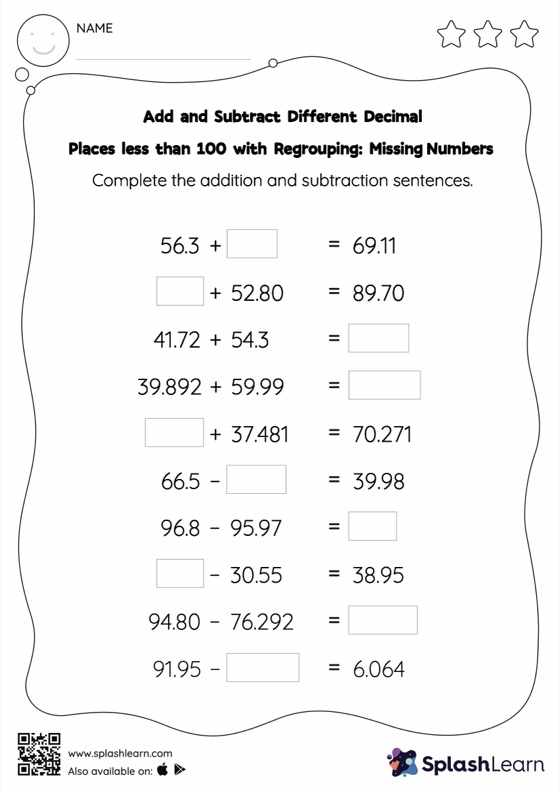# Add and Subtract Different Decimal Places less than 100 with Regrouping: Missing Numbers Worksheet

Home > Add and Subtract Different Decimal Places less than 100 with Regrouping: Missing NumbersStudents find the missing number while adding or subtracting decimals by using the relationship between addition and subtraction. To reach the answer, they also use the relationship between ones, tenths, hundredths, thousandths, etc., To regroup the numbers in add and subtract different decimal places less than 100 with regrouping worksheet. This worksheet is about practicing with the horizontal format in which numbers are written side by side. To develop flexibility with numbers and operations, students need to have enough practice in this format and not just rely on the vertical/column method.